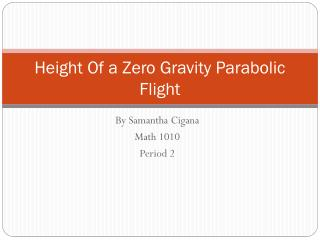DownloadDownload PresentationHeight Of a Zero Gravity Parabolic Flight

# Height Of a Zero Gravity Parabolic Flight

Download Presentation## Height Of a Zero Gravity Parabolic Flight

- - - - - - - - - - - - - - - - - - - - - - - - - - - E N D - - - - - - - - - - - - - - - - - - - - - - - - - - -
##### Presentation Transcript

1. Height Of a Zero Gravity Parabolic Flight By Samantha Cigana Math 1010 Period 2

2. Height Of a Zero-G t seconds after starting a parabolic flight path. To find the quadratic model, you will be plugging the data into the model h=at^2+bt+c. The data points given are just like x and y values, where the x value is the time t in seconds and the y value is the altitude h in feet. Plug these into the model and you will get equations with a b and c Time t in seconds Height h in feet

3. Part 1: write your 3 by 3 system of equations for a, b and c. 23645=4a+2b+c 32015=400a+20b+c 33715+1600a+40b+c For the first equation you square the first time to get a. Then b is 2 the time. And you set it all equal to the height which is 23645. you do this with all three.

4. Part 2: solve this system. Make sure to show your work. Step1: (23654=4a+2b-c)(-1) +32015=400a+20b+c 8361=396a+18b • You first take two of the three equations and do elimination. I took the first and times it by -1 and added the second and eliminated c. Step 2: 33715=1600a+40b+c +(32015=400a+20b+c)(-1) 1700=1200a+20b • In step two you repeat step one but choose two different equations. Step 3: (8361=396a+18b)(10) (1700=1200a+20b)(-9) = 83610=39600+180b -15300=-10800a-180b 68310= -6840a • In step three you take the answers to step one and two and eliminate b to find a.

5. Part 2 cont. • Step 4: 68310/-6840= • -6840/-6840= a=-9.987 • In step 4 you divide your answer in step for to get a. Step 5: 1700=1200(-9.987) + 20b -20b=1200(-9.987)-1700 -20b/-20=-13684.21/-20 B=684.21 • In step 5 you take one of the two equations from before and plug in a then set b equal to the whole equation and you get b. Step 6: 23654=4(-9.987+2(684.21)+c 39.948-1368.42+23654=c c=22325.528 • In the last step you plug in a and b then have c equal the equation and solve for c.

6. Part 3: using your solutions to the system from part 2 to form your quadratic model of the data -9.987x^2+684.21x+22325.528=p You use the equation a+b+c=p

7. Part 4: Find the maximum value of the quadratic function. -22325.528=-(x^2+684.22/9.987 x +1173.4) (x+34.26)+23525.928 Max value= 34.256 seconds Height= 34,045Ft For this part you put it in vertex form to find the height and max value.

8. Part 5: sketch the parabola Feet Seconds

9. Part 6: Reflective Writing • This is something used in everyday life. If I was ever going to be in the airplane industry this would be very useful. Using math that relates to everyday life helps me understand different concepts. I thought this project was very helpful and useful and was easy to understand new concepts.